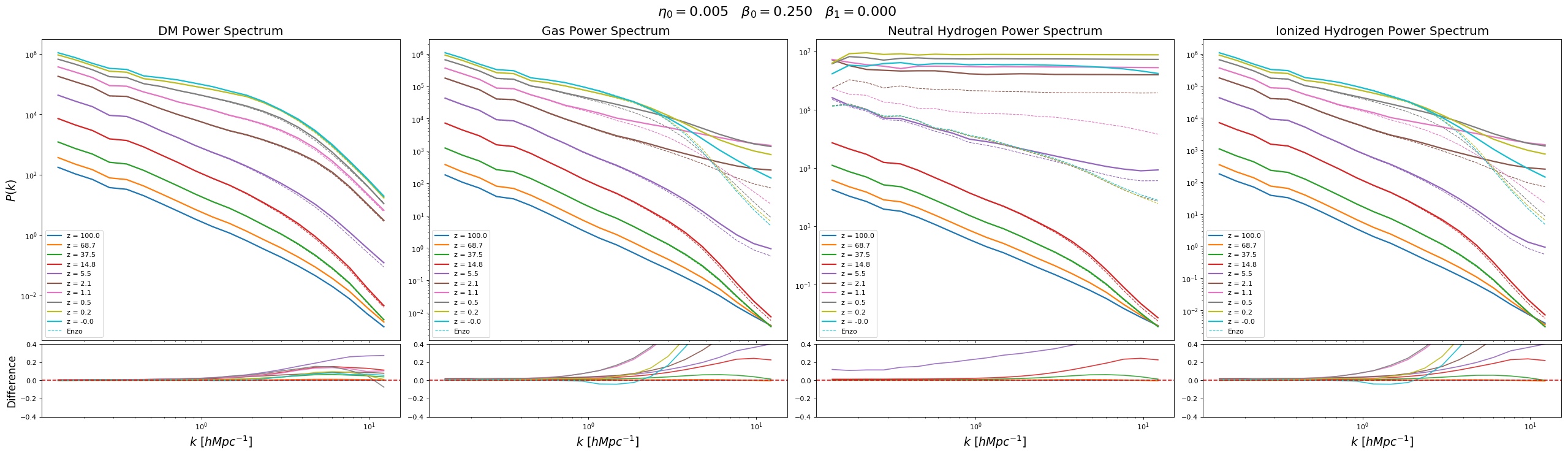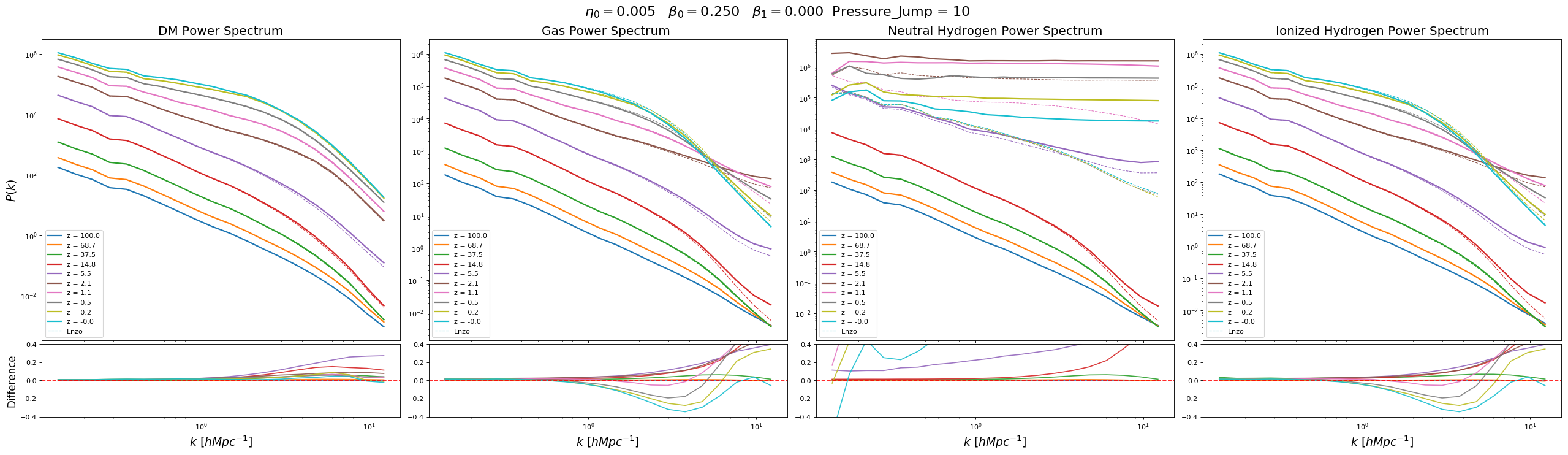The simulations are $128^3$ and 50Mpc.

Dual Energy Parameters: $\eta=0.005$ $\beta_0 = 0.25$ $\beta_1 = 0.0$

### Shock Detection

Pressure Jump:

From Fryxell 200 the Pressure Jump Condition for shock detection is:

Their implementation uses $\alpha = 0.1$

To ignore fluctuations due to noise a condition in the density is also applied:

### Phase Diagram

Row 1: Without Pressure Jump Condition

Now Using Pressure Jump condition:

Row 2: Using $\alpha=0.1$

Now only using the Total Internal Energy if $U_{total} > U_{advected}$

Row 3: Using $\alpha=1.0$

Row 4: Using $\alpha=10.0$

### Chemistry Projection

No Pressure Jump Condition

Using Pressure Jump Condition $\alpha=10.0$

### Power Spectrum

No Pressure Jump ConditionUsing Pressure Jump Condition $\alpha=10.0$